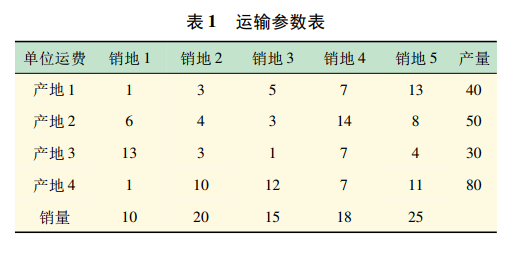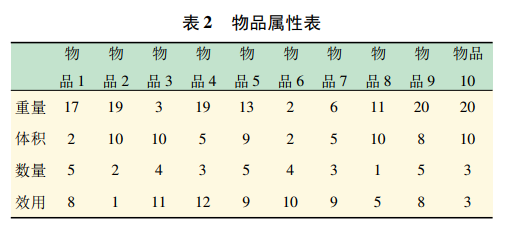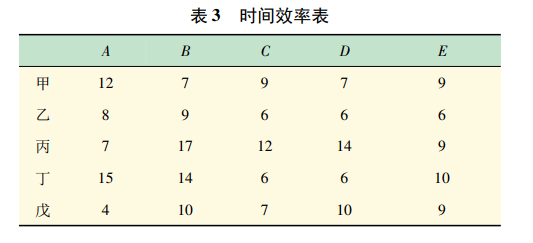• ## yalmip

2020-02-13 12:38:14
1、这个东西是用过，但是不经常用，还是...简书类 https://www.jianshu.com/p/e1c45b3d8d8a（Yalmip使用学习） https://www.jianshu.com/p/0f9cb5a29e47 （YALMIP + MOSEK） 博客园 https://www.cnblogs.com/kane19...
1、这个东西是用过，但是不经常用，还是会忘记的，因此还是做个总结。
2、先把其他人的资料收藏一下，参考 简书类 https://www.jianshu.com/p/e1c45b3d8d8a（Yalmip使用学习） https://www.jianshu.com/p/0f9cb5a29e47 （YALMIP + MOSEK） 博客园 https://www.cnblogs.com/kane1990/p/3428129.html（yalmip + lpsolve + matlab 求解混合整数线性规划问题（MIP/MILP）） csdn类 https://blog.csdn.net/qq_16309049/article/details/91549610(YALMIP学习笔记-基础知识) https://blog.csdn.net/s83625981/article/details/80076478(yalmip使用指南) 忘记了，官网的推荐 https://yalmip.github.io/
3、剩下的是自己的总结，等待中。。。
展开全文• ## YalMip

千次阅读 2017-02-02 21:10:48
https://yalmip.github.io/ ...YALMIP is free of charge to use and is openly distributed, but note that Copyright owned by Johan Löfberg. YALMIP must be referenced (general reference, robust op

https://yalmip.github.io/

YALMIP is free of charge to use and is openly distributed, but note that
Copyright owned by Johan Löfberg.  YALMIP must be referenced (general reference, robust optimization reference, sum-of-squares reference) when used in a published work (give me some credit for saving your valuable time!)  YALMIP, or forks or versions of YALMIP, may not be re-distributed as a part of a commercial product unless agreed upon with the copyright owner (if you make money from YALMIP, let me in first!)  YALMIP is distributed in the hope that it will be useful, but WITHOUT ANY WARRANTY, without even the implied warranty of MERCHANTABILITY or FITNESS FOR A PARTICULAR PURPOSE (if your satellite crash or you fail your Phd due to a bug in YALMIP, your loss!).  Forks or versions of YALMIP must include, and follow, this license in any distribution.
For installation, see the  installation tutorial, and get started coding  here
And don’t forget, most likely you have to  install additional solvers

https://github.com/yalmip/YALMIP/archive/master.zip

Installation
Updated: September 17, 2016
If it’s hard, you’re doing it wrong.

Getting started
Updated: September 17, 2016
Tutorial introduces essentially everything you’ll ever need. The remaining 95% is syntactic sugar.

Linear programming
Updated: September 17, 2016
As easy as it gets. Linear separation with linear norms.

Updated: September 17, 2016
Almost as easy as linear programming. Be careful though, symbolics might start to cause overhead.

Second order cone programming
Updated: September 17, 2016
Ice-cream cone! Yummy.

Semidefinite programming
Updated: September 17, 2016
Who wudda thought? Optimization over positive definite symmetric matrices is easy.

Determinant maximization
Updated: September 17, 2016
Optimization with ellipsoids and likelihood functions are typical applications of determinant maximization.

Duality
Updated: September 17, 2016
Extract dual solutions from conic optimization problems.

Sum-of-squares programming
Updated: September 17, 2016
Almost nothing is a sum-of-squares, but let’s hope yours is.

Robust optimization
Updated: September 17, 2016
The only thing we can be sure of is the lack of certainty.

Rank constrained semidefinite programming problems
Updated: September 17, 2016
Learn how to constrain ranks in semidefinite programs

Nonlinear operators - integer models
Updated: September 17, 2016
Mixed-integer representations of nonlinear operators

Nonlinear operators - graphs and conic models
Updated: September 17, 2016
Epi- and hypograph conic representations of nonlinear operators

Nonlinear operators - callbacks
Updated: September 17, 2016
Callback representations of nonlinear operators

Nonlinear operators
Updated: September 17, 2016
Working with nonlinear operators in a structured and efficient fashion

Multiparametric programming
Updated: September 17, 2016
This tutorial requires MPT.

Moment relaxations
Updated: September 17, 2016
Moment relaxations allows us to find lower bounds on polynomial optimization problems using semidefinite programming

Logic programming
Updated: September 17, 2016
Logic programming in YALMIP means programming with operators such as alldifferent, number of non-zeros, implications and similiar combinatorial objects.

Integer programming
Updated: September 17, 2016
Undisciplined programming often leads to integer models, but in some cases you have no option.

Global optimization
Updated: September 17, 2016
The holy grail! 60% of the time it works every time.

Geometric programming
Updated: September 17, 2016

General convex programming
Updated: September 17, 2016
YALMIP does not care, but for your own good, think about convexity also in general nonlinear programs.

Exponential cone programming
Updated: September 17, 2016
Convex conic optimization over exponentials and logarithms

Envelope approximations for global optimization
Updated: September 17, 2016
Outer approximations of function envelopes are the core of the global solver BMIBNB

Complex-valued problems
Updated: September 17, 2016
Complex data in optimization models. No problem in reality.

Bilevel programming
Updated: September 17, 2016
Bilevel programming using the built-in bilevel solver

Big-M and convex hulls
Updated: September 17, 2016
Learn how nonconvex models are written as integer programs using big-M strategies, and why it should be called small-M.

Automatic dualization
Updated: September 17, 2016

New release R20160930
Updated: September 30, 2016
Both patches and new features

Sample-based robust optimization
Updated: September 28, 2016
Unintended consequences of an improved optimizer framework

Extensions on the optimizer
Updated: September 28, 2016

MATLAB 2016 + CPLEX crash
Updated: September 23, 2016
Boom!

New release R20160923
Updated: September 23, 2016
It’s been a while…

Debugging infeasible models
Updated: September 22, 2016
Where to start?

Octave support in YALMIP
Updated: April 16, 2014
MATLAB no longer required! Recommended though.

Worst-case norms of matrices
Updated: February 08, 2014
Hard? Let’s try anyway.

Updated: June 27, 2013
Using YALMIP objects and code in Simulink models, easy or fast, your choice.

Unit commitment example - logic and integer programming
Updated: January 30, 2013
A common application of integer programming is the unit commitment problem in power generation, i.e., scheduling of set of power plants in order to meet a cu...

Updated: August 31, 2011
Common question: how can I solve a nonconvex QP using SeDuMi? Weird question, but interesting answer.

Strictly feasible sum-of-squares solutions
Updated: February 09, 2011
A question on the YALMIP forum essentially boiled down to how can I generate sum-of-squares solutions which really are feasible, i.e. true certificates?

Work-shop material
Updated: June 11, 2010
Files and exercise material from the YALMIP work-shop at the Swedish control conference 2010

Polytopic geometry using YALMIP and MPT
Updated: June 11, 2010
Ever wondered how to compute the L1 Chebyshev ball?

Tagging constraints
Updated: August 29, 2009
Name your constraints for easy reference

NaN in model
Updated: August 29, 2009
Where why how?

Computing multiple solutions in one shot
Updated: August 29, 2009
Avoid that for-loop by using vector objectives

Constraints without any variables
Updated: August 29, 2009
Code works for almost all cases, but suddenly fails.

New sum-of-squares example
Updated: August 29, 2009
Added a sum-of-squares example focusing on pre- and post-processing capabilities.


展开全文• yalmip_YALMIP例子_yalmip矩阵_简单例子_yalmip_YALMIP例子.zip
• yalmip_YALMIP例子_yalmip矩阵_简单例子_yalmip_YALMIP例子_源码.zip
• 求解线性矩阵不等式的简单例子，方便新手学习，yalmip工具箱教学
• yalmip_for_VRP_protectionska_yalmip遗传算法_vrp_cplex_yalmip.zip
• 基于matlab软件使用yalmip工具箱的一些微网的仿真、运行小例子
• matlab 的 yalmip 工具箱，方便进行优化问题的设置及求解优化
• 与遗传算法、蚁群算法等智能算法不同的是，yalmip工具箱调用CPLEX软件得到的解是精确解，且计算时间可以得到保证
• matlab波形优化算法经常要用到的matlab yalmip toolbox工具箱
• Untitled10_yalmip微网_Untitled10matlab_yalmip例子_微网_yalmip_源码.zip
• Untitled10_yalmip微网_Untitled10matlab_yalmip例子_微网_yalmip_源码.rar
• Hence it fails when it encounters any kind of YALMIP related code. In practice this means that all YALMIP code has to be placed in a so called Interpreted MATLAB function. This implies that you ...
• yalmip_for_VRP_protectionska_yalmip遗传算法_vrp_cplex_yalmip_源码.rar.rar
• 基于MATLAB和Yalmip的2变量机组组合调度算法
• The MATLAB toolbox YALMIP is introduced. It is described how YALMIP can be used to model and solve optimization problems typically occurring in systems and control theory.
• yalmip是一位“集大成者”，它不仅自己包含基本的线性规划求解算法，比如linprog（线性规划）、bintprog（二值线性规划）、bnb（分支界定算法）等，他还提供了对cplex、GLPK、lpsolve等求解工具包更高层次的包装。...
• YALMIP 求解LMI的另外一个重要工具 2014 10 30最新版本 注意新版本中set命令已被移除 因此 只需要使用加号或串联号来定义约束 如下 F [x > 0 x < 32]; F [F x^2 < 1]; F F + [y < 10 [x y;y 1] > 0];...
• 可以嵌套在MATLAB中，利用cplex求解，利用yalmip编程可以十分简单，更像自然语言
• ## yalmip简单的例子

万次阅读 多人点赞 2019-09-29 15:21:01
YALMIP应用篇 yalmip是一个在matlab内的建模工具包，能够用一套统一的建模语言来构建约束，调用其他的求解器，减少了单独学习其他语言的浪费，我根据论文 俞武扬. YALMIP工具箱在运筹学实验教学中的应用[J]. 实验室...
YALMIP应用篇
yalmip是一个在matlab内的建模工具包，能够用一套统一的建模语言来构建约束，调用其他的求解器，减少了单独学习其他语言的浪费，我根据论文

俞武扬. YALMIP工具箱在运筹学实验教学中的应用[J]. 实验室研究与探索, 2017(8).

由于该论文的代码有错误，我改写了一下 以下例子为论文中的例子 1.一般线性规划 2.运输问题 3.背包问题 4.指派问题 5.最短路问题

在写程序之前要把求解器如cplex，gurobi等路径设置好 然后用yalmiptest来测试一下是否能够调用求解器
yalmip基本格式
创建决策变量目标函数 z约束条件设置 C参数设置 ops = sdpsetting('solver','Cplex','verbose',0); verbose:显示冗余度 0为只显示结果求解 result = solvesdp(C,z,ops)
一般线性规划
model

min

⁡

Z

=

C

X

s.t.

{

A

X

=

b

X

⩾

0

\begin{array}{l}{\min Z=C X} \\ {\text { s.t. }\left\{\begin{array}{l}{A X=b} \\ {X \geqslant 0}\end{array}\right.}\end{array}

例子

min

⁡

Z

=

12

x

1

+

5

x

2

+

8

x

3

s.t.

{

2

x

1

+

3

x

2

+

x

3

⩾

30

4

x

1

+

x

2

+

5

x

3

⩾

15

x

1

,

x

2

,

x

3

⩾

0

\min Z=12 x_{1}+5 x_{2}+8 x_{3}\\ \text { s.t. }\left\{\begin{array}{l}{2 x_{1}+3 x_{2}+x_{3} \geqslant 30} \\ {4 x_{1}+x_{2}+5 x_{3} \geqslant 15} \\ {x_{1}, x_{2}, x_{3} \geqslant 0}\end{array}\right.

clear;clc;close all;

c = [12 5 8];
A = [2 3 1; 4 1 5];
b = [30; 15];

%决策变量
x = sdpvar(3,1);

%目标函数
z = c*x;

%添加约束
%C = [];
%C = [C; A*x >= b];
%C = [C;x>=0];
C=[A*x >= b,x>=0];
ops=sdpsettings('verbose',0);
%求解
result = optimize(C,z,ops);
if result.problem == 0    %求解成功
x_star=double(x)
z_star=double(z)
else
disp('求解过程中出错');
end

警告: 文件: C:\Program Files\IBM\ILOG\CPLEX_Studio_Community128\cplex\matlab\x64_win64\@Cplex\Cplex.p 行: 965 列: 0
在嵌套函数中定义 "changedParam" 会将其与父函数共享。在以后的版本中，要在父函数和嵌套函数之间共享 "changedParam"，请在父函数中显式定义它。
> In cplexoptimset
In sdpsettings>setup_cplex_options (line 617)
In sdpsettings (line 145)

x_star =

0
9.6429
1.0714

z_star =

56.7857

​ ​
运输问题
model

min

⁡

Z

=

∑

i

=

1

m

∑

j

=

1

n

c

i

j

x

i

j

s.t.

{

∑

j

=

1

n

x

i

j

⩽

a

i

,

i

=

1

,

2

,

⋯

,

m

∑

i

=

1

m

x

i

j

⩾

b

j

,

j

=

1

,

2

,

⋯

,

n

x

i

j

⩾

0

,

i

=

1

,

2

,

⋯

,

m

;

j

=

1

,

2

,

⋯

,

n

\min Z=\sum_{i=1}^{m} \sum_{j=1}^{n} c_{i j} x_{i j}\\ \text { s.t. }\left\{\begin{array}{ll}{\sum\limits_{j=1}^{n} x_{i j} \leqslant a_{i},} & {i=1,2, \cdots, m} \\ {\sum\limits_{i=1}^{m} x_{i j} \geqslant b_{j},} & {j=1,2, \cdots, n} \\ {x_{i j} \geqslant 0,} & {i=1,2, \cdots, m ; j=1,2, \cdots, n}\end{array}\right.例子
clear;clc;close all;

c = [1 3 5 7 13; 6 4 3 14 8; 13 3 1 7 4;
1 10 12 7 11];
a = [40 50 30 80];
b = [10 20 15 18 25];

%决策变量
x = intvar(4,5);

%目标函数
z = sum(sum(c.*x));

%添加约束
C = [];
for i=1:4
C = [C; sum(x(i,:))<=a(i)];
end
for j=1:5
C = [C;sum(x(:,j))>=b(j)];
end

C = [C;x>=0];
ops=sdpsettings('verbose',0);

result = optimize(C,z,ops);
if result.problem == 0    %求解成功
x_star = double(x)
z_star = double(z)
else
disp('求解过程中出错');
end

x_star =

2    20     0    18     0
0     0    10     0     0
0     0     5     0    25
8     0     0     0     0

z_star =

331

​ ​
背包问题
model

max

⁡

Z

=

∑

i

=

1

n

c

i

x

i

s.t.

{

∑

i

=

1

n

x

i

w

i

⩽

W

∑

i

=

1

n

x

i

v

i

⩽

V

0

⩽

x

i

⩽

n

i

且为整数

\max Z=\sum_{i=1}^{n} c_{i}x_{i} \\ \text { s.t. }\left\{\begin{array}{l}{\sum_{i=1}^{n} x_{i} w_{i} \leqslant W} \\ {\sum_{i=1}^{n} x_{i} v_{i} \leqslant V} \\ {0 \leqslant x_{i} \leqslant n_{i}\quad \text{且为整数}}\end{array}\right.

例子clear;clc;close all;

c = [8 1 11 12 9 10 9 5 8 3]; %效用
w = [17 19 3 19 13 2 6 11 20 20]; %重量
v = [2 10 10 5 9 2 5 10 8 10];  %体积
n = [5 2 4 3 5 4 3 1 5 3];   %数量

%决策变量
x = intvar(10,1);

%目标函数
z = -(c*x);

%添加约束
C = [];
C = [C,w*x<=80];
C = [C,v*x<=60];
C = [C,0<=x<=n];

ops=sdpsettings('verbose',0);
%求解
result = optimize(C,z,ops);
if result.problem == 0    %求解成功
x_star = double(x)
z_star = double(-z)
else
disp('求解过程中出错');
end

x_star =

1
0
3
1
0
4
3
0
0
0

z_star =

120

​ ​
最短路问题
% 利用yamlip求解最短路问题
clear;clc;close all;
n = size(D,1);

% 决策变量
x = binvar(n,n,'full');

% 目标
z=sum(sum(D.*x));
% 约束添加
C=[];
C = [C,(sum(x(1,:))-sum(x(:,1))==1)];
C = [C,(sum(x(n,:))-sum(x(:,n))==-1)];

for i=2:(n-1)
C = [C,(sum(x(i,:))-sum(x(:,i))==0)];
end

ops=sdpsettings('verbose',0);
% 求解
result=solvesdp(C,z,ops);
if result.problem == 0
x_star = value(x)
z_star = value(z)
else
disp('求解过程中出错');
end

x_star =

NaN     1     0     0     0     0     0
0   NaN     0     0     1     0     0
0     0   NaN     0     0     0     0
0     0     0   NaN     0     0     0
0     0     0     0   NaN     0     1
0     0     0     0     0   NaN     0
0     0     0     0     0     0   NaN

z_star =

5

​ ​
指派问题
model

min

⁡

Z

=

∑

i

=

1

n

∑

j

=

1

n

c

i

j

x

i

j

s.t.

{

∑

i

=

1

n

x

i

j

=

1

,

j

=

1

,

2

,

⋯

,

n

∑

j

=

1

n

x

i

j

=

1

,

i

=

1

,

2

,

⋯

,

n

x

i

j

∈

{

0

,

1

}

,

i

,

j

=

1

,

2

,

⋯

,

n

\min Z=\sum_{i=1}^{n} \sum_{j=1}^{n} c_{i j} x_{i j}\\ \text { s.t. }\left\{\begin{array}{ll}{\sum_{i=1}^{n} x_{i j}=1,} & {j=1,2, \cdots, n} \\ {\sum_{j=1}^{n} x_{i j}=1,} & {i=1,2, \cdots, n} \\ {x_{i j} \in\{0,1\},} & {i, j=1,2, \cdots, n}\end{array}\right.

例子clear;clc;close all;

%决策变量
x = binvar(5,5,'full');

%目标函数
z = sum(sum(c.*x));

%添加约束
C = [];

C = [C;sum(x,1)==1];   %  1 横向相加
C = [C;sum(x,2)==1];   %  2 纵向相加

ops=sdpsettings('verbose',0);
%求解
result = optimize(C,z,ops);
if result.problem == 0    %求解成功
x_star = double(x)
z_star = double(z)
else
disp('求解过程中出错');
end

c =

12     7     9     7     9
8     9     6     6     6
7    17    12    14     9
15    14     6     6    10
4    10     7    10     9

x_star =

0     1     0     0     0
0     0     0     1     0
0     0     0     0     1
0     0     1     0     0
1     0     0     0     0

z_star =

32

​ ​
展开全文• yalmip作为一门建模语言，其语言通俗易懂，容易上手，由可调用不同的外部求解器
• 适用于MATLAB进行优化计算，YALMIP工具箱yalmip是一个matlab的工具包，通过matlab实现各种操作和调用。 其次，它是一个建模工具，甚至可以称为一种“语言”，通过这种“语言”来描述模型，然后再调用其他求解器（如...
• 19年最新的YALMIP工具包。将压缩包解压到matlab/toolbox，在matlab主页界面上点击设置路径，选择 添加并包含子文件夹 将yalmip包中所有内容加入路径。matlab中调用yalmitest命令，查看是否添加完成。
• yalmip建模语言，可用于matlab，简化算法输入参数
• 1，将yalmip解压，在matlab中添加路径。 2，yalmiptest测试是否安装成功。
1，将yalmip解压，在matlab中添加路径。
2，yalmiptest测试是否安装成功。
展开全文...